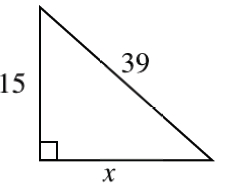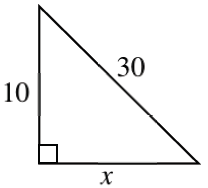### Home > INT3 > Chapter 2 > Lesson 2.2.4 > Problem2-108

2-108.

What is the value of $x$ in each diagram?

1.Recall the properties of the Pythagorean Theorem: $a^2+b^2=c^2$.

$(39)^2=(15)^2+c^2$

$1521-225=c^2$

$x=36$

1.Use the same method as (a)